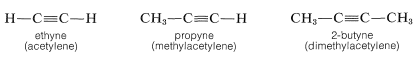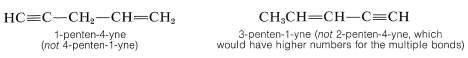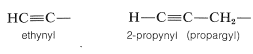# 3.5: Alkynes

$$\newcommand{\vecs}{\overset { \rightharpoonup} {\mathbf{#1}} }$$ $$\newcommand{\vecd}{\overset{-\!-\!\rightharpoonup}{\vphantom{a}\smash {#1}}}$$$$\newcommand{\id}{\mathrm{id}}$$ $$\newcommand{\Span}{\mathrm{span}}$$ $$\newcommand{\kernel}{\mathrm{null}\,}$$ $$\newcommand{\range}{\mathrm{range}\,}$$ $$\newcommand{\RealPart}{\mathrm{Re}}$$ $$\newcommand{\ImaginaryPart}{\mathrm{Im}}$$ $$\newcommand{\Argument}{\mathrm{Arg}}$$ $$\newcommand{\norm}{\| #1 \|}$$ $$\newcommand{\inner}{\langle #1, #2 \rangle}$$ $$\newcommand{\Span}{\mathrm{span}}$$ $$\newcommand{\id}{\mathrm{id}}$$ $$\newcommand{\Span}{\mathrm{span}}$$ $$\newcommand{\kernel}{\mathrm{null}\,}$$ $$\newcommand{\range}{\mathrm{range}\,}$$ $$\newcommand{\RealPart}{\mathrm{Re}}$$ $$\newcommand{\ImaginaryPart}{\mathrm{Im}}$$ $$\newcommand{\Argument}{\mathrm{Arg}}$$ $$\newcommand{\norm}{\| #1 \|}$$ $$\newcommand{\inner}{\langle #1, #2 \rangle}$$ $$\newcommand{\Span}{\mathrm{span}}$$

A number of hydrocarbons, called alkynes or acetylenes, have triple bonds between carbon atoms.$$^3$$ They conform to the general formula $$C_nH_{2n-2}$$ for one triple bond. The IUPAC system for naming alkynes employs the ending -yne instead of the -ane used for naming of the corresponding saturated hydrocarbon:The numbering system for locating the triple bond and substituent groups is analogous to that used for the corresponding alkenes:Hydrocarbons with more than one triple bond are called alkadiynes, alkatriynes, and so on, according to the number of triple bonds Hydrocarbons with both double and triple bonds are called alkenynes (not alkynenes). The chain always should be numbered to give the multiple bonds the lowest possible numbers, and when there is a choice, double bonds are given lower numbers than triple bonds. For example,The hydrocarbon substituents derived from alkynes are called alkynyl groups:$$^3$$Alkyne rhymes with "mine" and "thine."